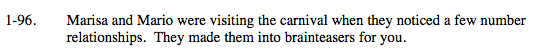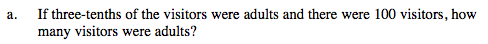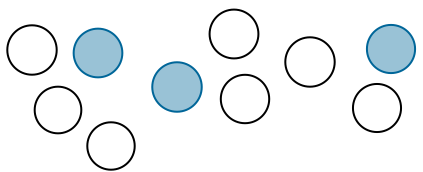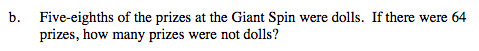### Home > MC2 > Chapter 1 > Lesson 1.2.4 > Problem1-96

1-96.If the shaded circles represent the fraction of adults, how many adults were present at the carnival?

Each circle is representative of 10 visitors.

There were 30 adults at the carnival.If the shaded boxes represent the fraction of prizes that were dolls, what fraction of the prizes were not dolls?

If there were 64 total prizes, how many prizes does each square represent?